• 1.实现一行输入多个数字，并以空格隔开。 a,b=map(int,input().split()) print(a,b) print(type(a)) #运行结果 1 2 1 2 2.实现一个输入多个单词，每个单词之间用逗号隔开。 str1,str2=map(str,input().split(','...输入
• Python3中实现一行输入多个数字并进行赋值

万次阅读 多人点赞 2019-03-26 22:20:19
Python3中实现一行输入多个数字并进行赋值1. input()和str.split()2. map(fun,seq)和列表推导式方法一：利用map(fun,seq)函数（推荐）方法二：利用列表推导式 参考学习后给出两个解决办法。 1. input()和str.split()...

Python3中实现一行输入多个数字并进行赋值

参考学习后给出两个解决办法。

1. input()和str.split()

首先input()函数返回的是一个字符串，如果你需要的不是数字，而是赋值字符串的话，你可以这样写：a,b,c = input().split()
具体解释如下

>>> a = input()   # input()返回的是字符串
121332
>>> a
'121332'  # 返回的是字符串
# 所以要多个赋值的话，需要对字符串进行分割，并返回列表，再进行赋值
# 我们采用str.split()方法进行分割，返回列表，如果不给出str.split()参数，默认是空格切割
>>> f = 'abcdabcdabcd'
>>> f.split('c')  # 以字符‘c'为切割标志，返回一个列表
['ab', 'dab', 'dab', 'd']
# 最后就是多重赋值了，a,b,c = ['1','2','3'] 相当于 a = '1'; b = '2'; c = '3'
>>> a,b,c = input().split()
1 2 3
>>> print(a,b,c)
1 2 3

2. map(fun,seq)和列表推导式

接下来解决我们的问题，要变为数字赋值，解决方案也比较简单，就是把字符串变为int。
这里给出两种方法：

方法一：利用map(fun,seq)函数（推荐）

a,b,c = map(int, input().split())
map(fun,seq)方法简单的说就是用fun这个功能函数去依次作用于序列seq中的每个元素，然后返回一个可迭代对象。

>>> a,b,c = map(int, input().split())
1 2 3
>>> print(a,b,c)
1 2 3

方法二：利用列表推导式

a, b,c = [int(x) for x in input().split()]
简单的解释这个列表推导式就是把input().split()返回的列表中的每一个元素通过遍历，依次用int()函数处理。

>>> a, b,c = [int(x) for x in input().split()]
1 2 3
>>> print(a,b,c)
1 2 3

注意以上的int,int()都可以用其他需要的数据类型替代，如float,float()等。

后记：
我从本硕药学零基础转行计算机，自学路上，走过很多弯路，也庆幸自己喜欢记笔记，把知识点进行总结，帮助自己成功实现转行。
2020下半年进入职场，深感自己的不足，所以2021年给自己定了个计划，每日学一技，日积月累，厚积薄发。
如果你想和我一起交流学习，欢迎大家关注我的微信公众号每日学一技，扫描下方二维码或者搜索每日学一技关注。
这个公众号主要是分享和记录自己每日的技术学习，不定期整理子类分享，主要涉及 C – > Python – > Java，计算机基础知识，机器学习，职场技能等，简单说就是一句话，成长的见证！展开全文input Python3
• Python 实现一行输入多个数字，用空格隔开

万次阅读 多人点赞 2019-03-29 11:10:58
很多人都会使用以下代码，来实现多个字符串的连续输入，...1.实现一行输入多个数字，并以空格隔开。 a,b=map(int,input().split()) print(a,b) print(type(a)) #运行结果 1 2 1 2 <class 'int'> 2.实现一...

很多人都会使用以下代码，来实现多个字符串的连续输入，但是这是以换行进行操作的，而有些题目需要将一些数字进行一行输入

a=input()
b=input()

1.实现一行输入多个数字，并以空格隔开。

a,b=map(int,input().split())
print(a,b)
print(type(a))

#运行结果
1 2
1 2
<class 'int'>

2.实现一个输入多个单词，每个单词之间用逗号隔开。

str1,str2=map(str,input().split(','))
print(str1,str2)
print(type(str1))

#运行结果
love,china
love china
<class 'str'>

例题：将二进制日期翻译为十进制的形式并输出。例如，日期 00010 00000 00010 00000 00001 00011，翻译为2020年1月3日。int(x,2)：将一个二进制的数字准换为十进制。

print("请输入报道日期，每位二进制数之间用空格隔开")
sstr=""
year1,year2,year3,year4,month,day=map(str,input().split())
sstr=sstr+str(int(year1,2))+str(int(year2,2))+str(int(year3,2))+str(int(year4,2))+"年"
sstr=sstr+str(int(month,2))+"月"
sstr=sstr+str(int(day,2))+"日"
print(sstr)

#运行结果
请输入报道日期，每位二进制数之间用空格隔开
00010 00000 00001 00100 00100 00001
2014年4月1日

展开全文• Python实现一行输入多个值

千次阅读 2021-03-09 15:33:57
1、输入多个值的方法：input()结合str.split()方法 需要注意：input()返回的是字符串类型，而str.split()方法返回的是列表类型 运行结果： 这里相当于字符串"22,55"经过split()方法，用","分割后返回的是列表[‘22...

1、输入多个值的方法：input()结合str.split()方法
需要注意：input()返回的是字符串类型，而str.split()方法返回的是列表类型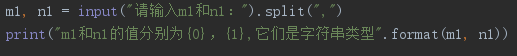运行结果：
这里相当于字符串"22,55"经过split()方法，用","分割后返回的是列表[‘22’, ‘55’]。其中m1=22、n1=55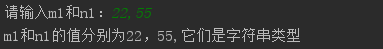2、输入多个值，将输入的值转换为整数
方法1：利用map(fun,seq)函数。下面的int可以用其他类型如float替代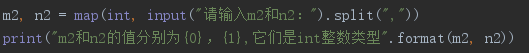运行结果：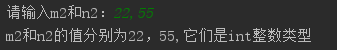方法2：直接利用列表推导式。下面的int可以用其他类型如float替代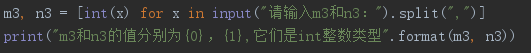运行结果：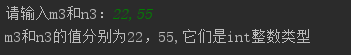-----end-----

展开全文python
• Python3在一行输入多个数字

万次阅读 多人点赞 2018-03-19 16:40:05
输入一个数字直接 m = int(input()) 输入个数字就是 m, n = map(int, input().split()) 三个及三个以上就和两个的类似:  a, b, c = map(int, input().split())  a, b, c, d = map(int, input().split()).....

输入一个数字直接 m = int(input())

输入两个数字就是 m, n = map(int, input().split())

三个及三个以上就和两个的类似:

a, b, c = map(int, input().split())

a, b, c, d = map(int, input().split())

展开全文• 编程题多个数相加计算程序任务内容:要求从键盘输入多个整数，数与数之间用逗号隔开，请计算出多个数之和。程序编写要求:（1）按要求使用不定长参数。（2）输出如下图所示：编程思路1、这里主要考察函数的不定长参数...
• python实现一行输入多个值和一行输出多个值

万次阅读 多人点赞 2019-01-11 18:49:26
一行输入多个值 a,b = input().split() #此时得到的a和b的类型均为字符串，以空格为分隔符 a,b = input().split(‘，‘) #此时得到的a和b的类型均为字符串，以逗号为分隔符 具体操作如下： a,b = map(int...python input output
• python如何一次性输入多个数

千次阅读 2021-02-04 01:05:45
python一次性输入多个数的方法：1、输入两个数字【m, n = map(int, input().split());】；2、输入三个及三个以上数字【a, b, c, d = map(int, input().split());】。python一次性输入多个数的方法：1、输入一个数字...
• Python 之 如何一行输入多个整数 例如：在一行 输入 a,b 以字符串形式保存 a,b = input().split() 以整数形式保存 a,b = map(int,input().split()) 输入多个数字也是类似，以整数形式保存： a,b,c = map(int...python
• Python2.7在一行输入多个数字

千次阅读 2018-06-22 20:34:42
输入一个数字 m = int(raw_input())输入个数字是 m, n = map(int, raw_input().split())三个及三个以上类似: a, b, c = map(int, raw_input().split()) a, b, c, d = map(int, raw_input().split())...python
• python一行输入n个数据

万次阅读 多人点赞 2019-11-22 21:18:05
有时会碰到一行输入多个数据，这是可以先用str类型存一组数据，然后再迭代的将每个数据追加到新的列表中。 方法一：先输入str字符串，再根据空格得到数字字符列表n.split（）.然后将列表元素转为数字存到新的...
• #第一行将整一行输入值放入列表lis中，注意，由于input函数的作用，每一列表元素都是str类型 for i in range(len(lis)): lis[i] = int(lis[i]) #将每一列表元素的类型从str转换为int型 ...
• python一次性输入多个数的方法发布时间：2020-09-22 09:29:29来源：亿速云阅读：75...python一次性输入多个数的方法：1、输入一个数字直接m = int(input())2、输入两个数字就是m, n = map(int, input().split())3...
• Python-一行输入多个数，并存入列表

万次阅读 多人点赞 2020-05-19 11:08:19
• python实现input一行输入多个值

万次阅读 多人点赞 2019-04-27 14:59:21
如果想传入多个值，我们可以使用字符串的spilt函数，以空格进行字符串的分割，并返回个列表。如下所示： a,b = (input("请输入两个单词：").split()) print(a,b) 请输入两个单词：hello word hello word 上面...
• python怎么一次输入两个数

千次阅读 2020-12-29 12:06:26
Python输入多个数字：a, b, c = map(int, input().split())1、输入个数字直接 m = int(input())2、输入两个数字就是 m, n = map(int, input().split())3、三个及三个以上就和两个的类似:a, b, c = map(int, ...
• 我就废话不说了，大家还是直接看代码吧~n = input("1st enter:")print(n)print(type(n))n = int(input("2nd enter:"))print(n)print(type(n))n, m, c = input("3rd enter:")print(n, m, c)print(type(n))n, m, c =...
• 这里要使用一个函数 input().split() 如果使用 eval(input()) 会报错 下面给出一个例子 a,b,c=input().split() #输入整数 b=int(b) #进行强制类型转换 c=int(c) a=int(a) ans=b*b-4*a*c #计算方程结果 print...python
• 输入多个数字（这里以5个为例），然后将数字放到列表中，并且按照从小到大排序下面我们看下如何用python实现这个功能工具/原料电脑python开发工具方法/步骤1在Python开发工具中新建个空白的Python文件，名称为数字...
• Python输入一行任意个数的整数

万次阅读 多人点赞 2019-08-08 16:07:34
Python输入一行任意个数的整数 Python作为一种强大快捷的语言，近几年是越来越火，...Python读入多行输入数字是没问题的，但是读入一行多个数字可能有些棘手。 话不多说，上题（选取SDUTACM oj上的题1147）： 初学...
• 1. 例如：在一行 输入 a,b  以字符串形式保存 a,b = input().split() 2. 以整数形式保存 ...a,b = map(int,input().split())...输入多个数字也是类似：以整数形式保存： a,b,c = map(int,input().split())   ...
• 一行输入多个整数。例如，用户在键盘敲入的一行有4个整数： 3 44 8 99，程序该如何接收这4个整数？ 在一行输入多个浮点数。例如，用户在键盘敲入如下一行内容： 2.5, 7.8, 1.1，这一行有3个浮点数。程序该怎么...
• 今天小编就为大家分享Python 实现输入任意多个数,并计算其平均值的例子，具有很好的参考价值，希望对大家有所帮助。一起跟随小编过来看看学习了Python相关数据类型，函数的知识后，利用字符串的分割实现了输入......

python一行输入多个数字python 订阅